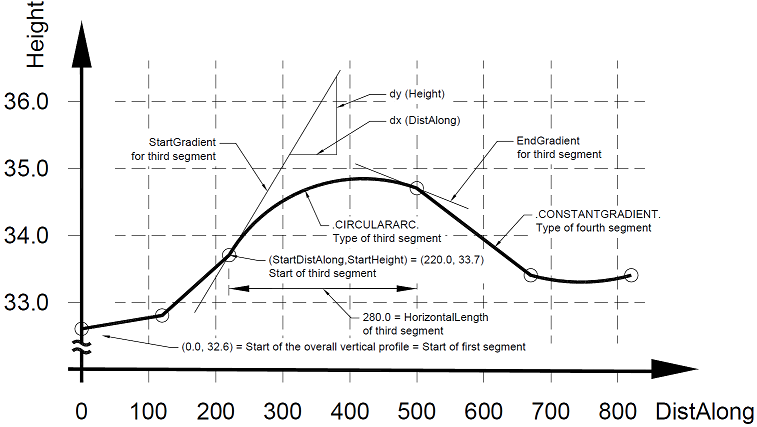# IFC 4.3.x development

## 8.7.3.7 IfcAlignmentVerticalSegment

### 8.7.3.7.1 Semantic definitions at the entity

Individual segment along the IfcAlignmentVertical, being defined in the distance-along/z coordinate space.

The vertical alignment is defined by segments that connects end-to-start. The vertical alignment curve geometry is defined in a plane with x = distance along horizontal, the y = height (or elevation). The transition at the segment connection is not enforced to be tangential, The IfcSegment Transition enumeration specifies the type of transition explicitly.

The following vertical segment types are defined:

For each vertical segment, the following non-redundant information is provided:

• the start point (in distance along/ height coordinates)
• the start gradient (as a ratio measure with horizontal being 0, uphill positive, and downhill negative) usually between 1 < n < -1 (equal to a percentage of 100% < n < -100%, or to a degree of 45° < n < -45° but higher values are possible)
• the length (as horizontal length along the distance along (not the curve segment length))
• the curve parameter needed for circular and parabolic arc segments

The following information can be calculated (and is therefore not exchanged explicitly to avoid redundancy and inconsistencies)

• the end distance along (from the distance along and segment length)
• the end height (from start distance along, gradient, length and curve parameter)
• the end direction (from start direction, segment length and curve parameter)
• the point of vertical intersection (from start direction and end direction)

The following checks can be done to validate the correct exchange:

• continuity – does the calculated end distance along of the previous segment matches with the provided start distance along of this segment
• tangential continuity – does the calculated end gradient of the previous segment matches with the provided start gradient of this segment

NOTE  Specific subtypes of the IfcAlignmentVerticalSegment add specific geometric curve parameters. Connectivity between vertical segments is not necessarily tangential, but this can be enforced as a requirement through the attribute TangentialContinuity.### 8.7.3.7.1 Attributes

#Attribute Type Description
IfcAlignmentParameterSegment
1StartTag OPTIONAL IfcLabel Tag to annotate the start point of the alignment segment.
2EndTag OPTIONAL IfcLabel Tag to annotate the end point of the alignment segment.
IfcAlignmentVerticalSegment
3StartDistAlong IfcLengthMeasure Distance along the horizontal alignment as measured along the corresponding IfcAlignmentHorizontal.

> NOTE 1  The distance along is measured from the start point of IfcAlignmentHorizontal, any optionally provided offset expressed by IfcAlignmentHorizontal.StartDistanceAlong is not taken into account.

> NOTE 2  The unit of measurement is the global length unit, as set by IfcContext.UnitInContext
4HorizontalLength IfcPositiveLengthMeasure Length measured as distance along the horizontal alignment of the segment.
5StartHeight IfcLengthMeasure Elevation in Z of the start point relative to the IfcAlignment coordinate system.

> NOTE  It is strongly advised to not offset the IfcAlignment coordinate system from the project engineering coordinate system.
8RadiusOfCurvatureOPTIONAL IfcPositiveLengthMeasure Radius of parabola or arc. Positive values imply a CCW direction whereas negative CW.

> NOTE1  For PredefinedType is ARC. The radius of the basis circle for the arc.

> NOTE2  For PredefinedType is PARABOLICARC. Parabola constant (determining the “steepness” of the parabola). The parabola constant is provided by the “minimum parabola radius”, the true radius of a parabola at its vertical axis (the zero-gradient point of the parabola). The minimum radius is twice the focal length of the parabola (the distance between the focal point and the vertex).

> NOTE3  For PredefinedType that is not either ARC or PARABOLICARC the value should be empty.
9PredefinedType IfcAlignmentVerticalSegmentTypeEnumPredefined type of the vertical alignmnent segment.

### 8.7.3.7.3 Formal representations

```ENTITY IfcAlignmentVerticalSegment
SUBTYPE OF (IfcAlignmentParameterSegment);
StartDistAlong : IfcLengthMeasure;
HorizontalLength : IfcPositiveLengthMeasure;
StartHeight : IfcLengthMeasure;